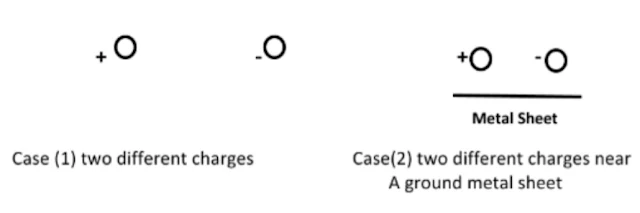# Fist Year Power Engineering: Electromagnetic Fields Sheet (2) Static Electric and Magnetic Fields

Fist Year Power Engineering:   Electromagnetic Fields
Sheet (2)
Static Electric and Magnetic FieldsStatic Electric and Magnetic Fields

1-Coulomb’s  Law and the electric field E:

1.1: define coulomb’s law, mention units .
1.2 : define the electric field strength E , derive E from coulomb’s law.
1.3: solve the following field problem:
Calculate the electric field intensity E at the point C (2,1,4)m due to point charges at:
A:6μC at (3,1,3)m and - 8μC at    B: (1,-1,2)m.

2-The Electric Potential V:

2.1 define the electric potential difference between two points and the absolute
Potential of a point.
2.2 derive the formula for absolute potential of a point due to a point charge.
2.3 as E=- grad V  and D= ε E  solve the following problems:
A: Calculate :
The E at the center of a rectangle of dimensions 8 x 6 m due to charges:
Qa = Qb = 6 μC and Qc = Qd = - 10μC
B: If the potential at any point V(x,y,z) is given by V(x,y,z)= 3x2 y3 z-1     V,
calculate: (for the medium with εr = 40 ):
At the point (2,5,1) m , the electric field intensity E – the electric flux density D
C: Calculate :
FOR : a charged  Ring in X-Y plane  Fig (1) with radii: inner  a= 2 and outer
b= 2.1 m  has a total charge   5pC    . Consider a  narrow ring and a  free       z
space  medium.

(a): the surface charge density over the ring
(b): the electric potential V at the point P(0,0,10)m            Fig(1)

3-The Field Lines Due to Charges:

Use your knowledge to map the field lines between the following charges:Metal Sheet               Case (1) two different charges                      Case(2) two different charges near A ground metal sheet